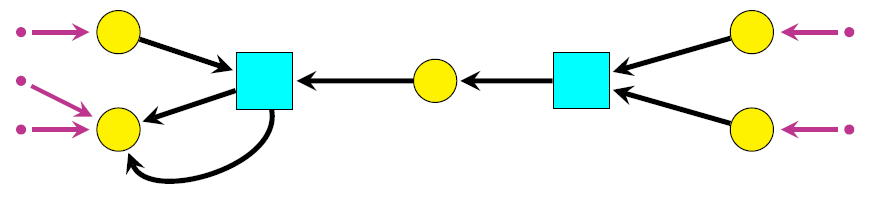#### John BaezHere are two related talks on structured cospans.

## Structured Cospans and Double Categories

One goal of applied category theory is to better understand networks appearing throughout science and engineering. Here we introduce “structured cospans” as a way to study networks with inputs and outputs. Given a functor $$L \colon A \to X$$, a structured cospan is a diagram in $$X$$ of the form

$$L(a) \to x \leftarrow L(b)$$

If $$A$$ and $$X$$ have finite colimits and $$L$$ is a left adjoint, we obtain a symmetric monoidal category whose objects are those of $$A$$ and whose morphisms are certain equivalence classes of structured cospans. However, this arises from a more fundamental structure: a symmetric monoidal double category where the horizontal 1-cells are structured cospans, not equivalence classes thereof. We explain the mathematics and illustrate it with an example from epidemiology.

You can see the slides here, download a video here, or watch the video here:

This talk is based on work with Kenny Courser and Christina Vasilakopoulou, some of which appears here:

## Structured Cospans and Petri Nets

“Structured cospans” are a general way to study networks with inputs and outputs. Here we illustrate this using a type of network popular in theoretical computer science: Petri nets. An “open” Petri net is one with certain places designated as inputs and outputs. We can compose open Petri nets by gluing the outputs of one to the inputs of another. Using the formalism of structured cospans, open Petri nets can be treated as morphisms of a symmetric monoidal category — or better, a symmetric monoidal double category. We explain two forms of semantics for open Petri nets using symmetric monoidal double functors out of this double category. The first, an operational semantics, gives for each open Petri net a category whose morphisms are the processes that this net can carry out. The second, a “reachability” semantics, simply says what these processes can accomplish.
You can see the slides here or watch a video here:

The talk is based on joint work with Kenny Courser and Jade Master: